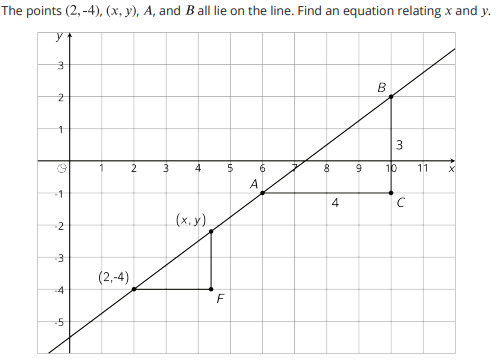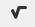PSABM4ZV - Practice---Acc7.3 Lesson 11 Using Equations to Solve Problems (7.EE.B.3, 7.EE.B.4, 7.EE.B.4.a)
Part A)

Match the equation to its solution.

5x3

x = _?_

Select one:
Part B)

3(5+x)

x = _?_

Select one:
Part C)

3-7

x = _?_

Select one:
Part D)(x+75

x = _?_

Select one:
Part A)

Match each equation to the story it describes.

5x-7 = 3

Select one:
Part B)

7 = 3(5-x)

Select one:
Part C)

3x + 5 = -7

Select one:
Part D)(x+75

Select one:

The baby giraffe weighed 132 pounds at birth. He gained weight at a steady rate for the first 7 months until his weight reached 538 pounds. How much did he gain each month?

Type your answer below as a number (example: 5, 3.1, 4 1/2, or 3/2):
Part A)

Six teams are out on the field playing soccer. The teams all have the same number of players. The head coach asks for 2 players from each team to come help him move some equipment. NOW there are 78 players on the field. Write an equation whose solution is the number of players on each team.

Part B)

Type your answer below as a number (example: 5, 3.1, 4 1/2, or 3/2):Enter your equation using the WIRIS editor button